# Vectors

Vector addition and subtraction, the scalar product (dot product) and the vector product (cross product). This page supports various multimedia tutorials in Physclips, including initially Constant Acceleration and Circular Motion.

###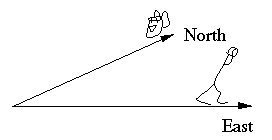A vector has magnitude and direction

Let's start with displacement, which is a vector. That means that it is specified by both a magnitude and a direction. If I move my bag 10 metres North, it is not in the same position as it would be if I were to displace it 10 metres East - I'd certainly notice the difference when I went to look for my bag.

The magnitude of the displacement is just how far the object is from the origin of reference – 10 m in my examples above. The direction may be specified in any convenient way. Here are some examples of vectors:

• 10 metres North (a displacement)
• 15 kilometres per hour, directly towards the opponent's goal (a velocity), and
• 9.8 m.s−2 down (an acceleration).

Directions are sometimes expressed in terms of North and South, East and West, up and down. We might also use "radially outwards from the centre of the circle" or "parallel to the initial direction" etc. In very many physics problems, we define a system of axes and use them.

To distinguish a vector, some books used bold face. In handwriting, we usually use underlining. Here we use both. The symbol r is commonly used for a displacement, just as v is used for velocity and a is used for acceleration.

### An example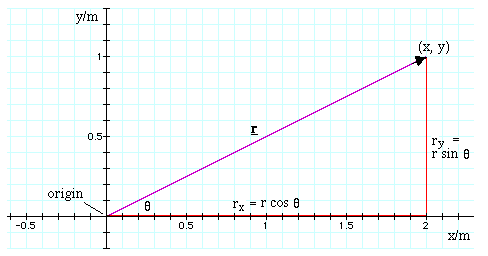Let's consider a displacement in the x,y plane. Suppose I displace an object from the origin (0,0), to the point (2.0,1.0), where the units are metres. The purple arrow shows this displacement. We'll call this displacement r.

Magnitude and direction: How far have we moved it? From Pythagoras' theorem, the distance moved is ((1.0 m)2 + (2.0 m)2)½ = 2.2 m. In what direction have we moved it? We could describe this in several ways, including this: at angle θ from the x axis (in the positive mathematical sense). From trigonometry, tan θ = 1.0/2.0 so θ = 27°. In other words, we could describe it as

r  =  2.2 metres at +27° from the x axis.
If our y and x axes were the North and East on a map, we should similarly say r  =  2.2 metres at +27° North of East.

Note that, in both cases, we have given a magnitude and direction. This is necessary: because r is a vector, it has magnitude and direction. There are two pieces of information on the left hand side of the equation and so must there be on the right.

To write the magnitude of a vector, we simply use normal type face. In this example, note carefully the difference

r  =  2.2 metres,
but
r  =  2.2 metres at +27° from the x axis.
Sometimes we may write the magitude of a vector like this: r  =  |r|   (=  magnitude of r).

### Components

The two red lines in this triangle have already been useful, and they have more work to do. Suppose that I displace an object 2.0 metres in the x direction (the horizontal red line), then displace it 1.0 metre in the y direction (the vertical red line). It ends up at the point (x,y) = (2.0 m,1.0 m). So the two red lines are displacements that add up to give the purple line. We could say that r = (2.0 m in the x direction) plus (1.0 m in the y direction). We say that r has an x component and y component and, using the definition of sin and cos:
rx  =   2.0 m   =   r cos θ   and
ry  =   1.0 m   =   r sin θ.
These two equations tell us how to calculate the components of a vector, given its magnitude and direction. We need not restrain ourselves to the x and y axes: we can do this in any two or more perpendicular directions. This is a very important technique in analysing the forces acting on an object: we often wish to consider the forces acting in the direction of motion and at right angles to it.

To obtain the magnitude and angle from the components, we can use Pythagoras' theorem and the definition of the tan function, respectively, to give us:

r  =   (rx2 + ry2)1/2    and
θ  =  tan−1 (ry/rx).
Note that the components rx and ry are scalars, so we cannot simply add them to get r, which is a vector. To add rx and ry, we need to specify their directions. We do this using unit vectors.

### Unit vectors

If we say "Go 2.0 metres East, then 1.0 metre North", what does "East" mean? It tells us just direction, so does it have a magnitude? Suppose we give "East" a magnitude of one (not one metre, just one). Now we can treat "2.0 metres East" as a product. In this sense, East is a unit vector.We reserve the vectors i, j and k as unit vectors in the x, y and z directions, respectively. Now we can write:
r  =  ri + rj    =  (2.0 m)i + (1.0 m) j
In other words, the displacement r may be achieved by going a distance rx in the i (or x) direction, plus a distance ry in the j (or y) direction. If that looks strange, then let's consider the unit vectors N for North and E for East. Translate "Go 2.0 metres East, then 1.0 metre North" to give (2.0 E + 1.0 N) metres.

Of course, we don't need to use unit vectors in all circumstances. We could say "in the positive x direction" instead of i. However, apart from brevity, the advantage of unit vectors is that we can now do vector algebra very simply, as we shall see in the sections on addition, subtraction and multiplication of vectors.

Incidentally, you may also see unit vectors in circular, cylindrical or spherical polar coordinates. These include a unit vector in the radial or r direction and unit vectors in the θ, φ and/or z direction. However, we don't use these in the Mechanics section of Physclips.

### Vectors in three dimensionsIn an (x,y,z) coordinate system, we shall use all three unit vectors of the Cartesian system and write, for a vector a: a  =  ax i + ay j + az k. There are two different ways in which the positive z axis could be at right angles to the postive x and y axes. By convention, we choose what is called the right-handed system: If your right thumb and index finger point in the direction of x and y, then it is possible to point your right middle finger in the direction of z, as shown in the sketch. (Apologies for the sketch.) A useful result comes from an extension of Pythagoras' theorem to three dimensions, which is also shown in that diagram. For the vector a, what is the magnitude a? First, we can use Pythagoras for the hypotenuse h and write: h2  =  ax2 + ay2. Now look at the triangle that has a as its hypotenuse. For this triangle, a2  = h2 + az2. Substitute for h from the first of these two equations into the second and we have Pythagoras in three dimensions, and thus means of calculating the magnitude of a vector with three Cartesian components: a2  =  ax2 + ay2 + az2. What about four dimensions? What if we want the spacetime separation of two events at (x1,y1,z1,t1) and (x2,y2,z2,t2)? This takes us to special relativity, which we introduce here. Briefly, we'd write a2 = (x22 − x12) + (y22 − y12) + (z22 − z12) − (ct22 − ct12).First note that, on this diagram, two arrows are labelled b. However, each arrow has the same magnitude and the same direction, therefore they are the same vector. Now let's think of vectors a and b in this diagram as displacements. Let's say I move through the displacement a (ie, I travel from the tail of a to its head). To add a displacement b to this, I shall move through b, starting from where I have just arrived, at the head of a. In other words, I put the two vectors head to tail. So, in this diagram,
a + b  =  c.
The order doesn't matter: if I duplicate a and put its tail at the head of b, I also get c, as you can see by mentally completing the parallelogram. So let's note that
b + a  =  c  =  a + b,
which is not as trivial as it seems.

To quantify the addition, one could use geometry. However, if we know the components (or if we can find them) it's often easier to write:

c  =  a + b  =  (ai + aj) + (bi + bj) ,    so

ci + cj =  (a + b)i + (a + b)j.

Now the last equation gives us an expression for the x component of c -- and also for its y component. In other words a vector equation in two dimensions gives us two scalar equations. c  =  a + b gives us:
c  =  a + b    and

c  =  a + b.

This is important to remember when you are 'chasing an extra equation' -- ie when you think that you have only n equations to solve for n+1 unknowns! And of course in three dimensions a vector equation gives three scalar equations.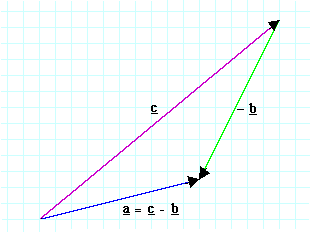#### Subtracting vectors

Let's take our previous example and rearrange
a + b  =  c,
to obtain
a  =  cb.
We expect that bb = 0. In other words, after a displacement b, − b must bring us back to where we started. So the vector − b has the same magnitude as b, but the opposite direction. In this diagram, we have therefore drawn
cb  =  c + (− b).
It is also helpful to look at these two diagrams and to remind yourself that (c − b) is the vector that you must add to b in order to get c. In other words, it is the vector a of the previous diagram.

### Relative motion and moving coordinates

Relative motion is a good source of applications of vector addition and subtraction, so let's do some here. Let's start with an easy case, which you will already know and do the more formal derivation later.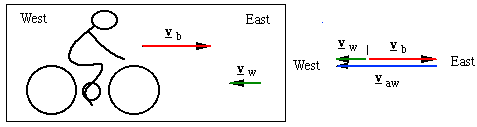#### What does a head wind feel like?

Suppose that the wind is coming from the East (ie towards the West), at 5 m.s−1. So the velocity of the wind, relative to the ground, is vw = 5 m.s−1 West. If you are bicycling East at 10 m.s−1 (ie vb = 10 m.s−1 East), what will be the velocity of the wind, relative to you? How strong is the wind that you feel on your face?

The velocity of the wind with respect to the ground, vw is usually called the true wind, and the velocity relative to an observer moving over the ground is called the apparent wind, vaw. So this question would often be expressed thus: what is the apparent wind? Or, alternatively, what is the velocity of the wind, vaw, in a coordinate system moving with you?

Here, it's easy: the wind is travelling at vaw with respect to you, and you are travelling at vb with respect to the ground, so the true wind is:

vw  =  vaw + vb .     So:
vaw  =  vwvb.
In the case of the cyclist going East and the wind going West, we could write the wind's velocity as
vw  =  5 m.s−1 West  =  − 5 m.s−1 East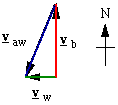#### What does a cross wind feel like?

What if you are travelling North, with the wind from the East, with the same speeds as before? What will be the apparent wind then?

The same colour coding is used as in the previous example. Using the right angle triangle, we calculate the angle at the top: it is tan−1vw/vb. Note that, if vw < vb, which is often the case, the apparent wind due to a side wind can be reasonably close to an apparent head wind, as is shown here.

Cyclists often say "Tailwinds are rare, and downhill tailwinds don't happen." Using vector subraction, can you explain this belief?

Addition and subtraction of velocities is also very important to sailors, first because the velocity of the boat over the water adds to that of the water over the ground to give the boat's velocity with respect to the ground. More importantly, the relative velocity of the wind, as measured on the boat, is the 'true wind' (ie the velocity of the wind with respect to the land) minus the velocity of the boat with respect to the land. See The physics of sailing for examples. Also see Plane on a conveyor belt.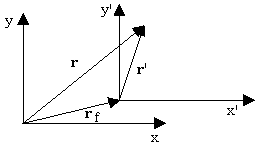### Position, velocity and acceleration in different frames

Let's derive that more generally: suppose that we measure position r in a frame of reference fixed on the ground. Let the origin of a frame moving at constant velocity with respect to the ground be rf with respect to the frame fixed on the ground. So the velocity of the moving frame is vf = drf/dt. Let position in the moving frame be r', so

r  =  rf + r' .
Provided that time can be measured by synchronised clocks in both frames (but see Relativity for details), we can take derivatives of both sides
v  =  dr/dt  =  drf/dt + dr'/dt  = vf + v' ,    or      v'  =  v − vf ,

where v' is the velocity measured in the moving frame (called the dashed frame). Taking time derivatives again
a  =  dv/dt  =  dvf/dt + dv'/dt  = af + a' ,
where a' is the acceleration measured in the moving frame. However, we assumed that the moving frame had a constant velocity, so af is zero, so
a = a
If we observe the same forces in both frames, we see that, subject to the assumptions we've noted, the accelerations are the same and so the laws of mechanics are the same. This brings up the topic of relativity, in both Galilean and Einsteinien form, which we discuss in much greater detail in this link.

### The scalar product (the dot product)

What does the product of two vectors mean? It must obviously be rather different to the product of two numbers. The answer is that it could mean anything that we define it to mean, provided that the definition is consistent. There would be no point in defining the product unless it were useful, so let's see where we could use it.
Work gives us one example. When the point of application of a force F (a vector) has displacement s (another vector), the the work done (a scalar) is proportional to F and it is also proportional to s. So there is a good argument for defining the product of vectors so as to get a scalar. Now the work done also depends on the angle θ between F and s, in fact:
W  =  F.s.cos θ.
This is one reason why we define the scalar product or the dot product of two vectors thus:
a.b  ≡  a.b.cos θ
where θ is the angle between a and b.
It is called the scalar product because, as you can see, the right hand side is a scalar. It is also called the dot product, because the dot between a and b signifies multiplication thus defined. We pronounce it "a dot b". Let's look at a few corollaries of this definition.
a.a  ≡  a.a.cos 0  =  a2
ie the scalar product of a vector with itself is its magnitude squared. If we take the dot product of two vectors at right angles to each other, the expression includes the cosine of a right angle, which is zero. For reasons that will become clear below, we should note an important result that is less trivial than it looks:
a.b  =  b.a
We can apply these observations immediately to unit vectors (their magnitude is one, remember), to obtain these important results:
i.i  =  1     and similarly      j.j  =  k.k  =  1,     while

i.j  =  j.k  =  k.i  =  0.

This allows us to simplify algebra somewhat. Take the two vectors
a  =  ai + aj + ak      and
b  =  bi + bj + bk
We can now write their scalar product in two different ways:
a.b  =  a.b.cos θ      and
a.b  =  (ai + aj + ak).(bi + bj + bk)
=  ab + ab + ab.
Setting these last two results equal, by the way, gives a really neat way of working out the angle between two vectors. (And if you don't think that this is neat, try working out θ some other way!) You can also use the dot product to calculate the components of vectors, because, as you can see from applying the results above,
a.i  =  a     and so on for the other components.

### The vector product (the cross product)

We've just seen that the scalar product (or dot product) of two vectors was a scalar. The vector product (or cross product) is – you've guessed already. First, here are a couple of examples where we need it.
Consider the magnetic force F on a charge q travelling at speed v in magnetic field B. (See electric motors for examples of magnetic forces.) The magnitude of that force is proportional to v and proportional to B, so we need a product of the two vectors. The magnitude F of the force is also proportional to sin θ, where θ is the angle between v and B. Now this magnetic force F is a vector, so we need not just its magnitude, but its direction. That direction is at right angles to both v and B.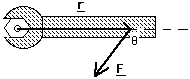Now consider the torque τ produced by a force F about an axis from which it is displaced by r. (See the Rotation chapter on Physclips.) The magnitude of that torque is proportional to r and proportional to F, so again we need a product of two vectors. The magnitude τ of the torque is also proportional to sin θ, where θ in this case is between r and F. (Notice that it, in both these cases, we need sin θ, whereas the scalar product had cos θ). The torque τ is a vector: torques in different directions in general cause rotations about different axes. That direction of the torque (which is often but not always parallel to the axis about which it causes rotation) is at right angles to both r and F.So we define the vector product thus: |a X b|  =  ab sin θ, and a X b is at right angles to a and b in a right handed sense. a X b is pronounced "a cross b".The "right handed sense" is needed because there are two directions at right angles to a and b. So, if the thumb of your right hand is in the direction of a and your right forefinger in the direction of b, then your right middle finger is in the direction of a X b. Unless your hands are extraordinarily flexible, the thumb and two fingers can only be mutually perpendicular in one way. Another way to define the direction, which doesn't require hands, is that, if the plane of a the blade of a screwdriver is rotated from a to b, then a normal (right-handed!) screw advances in the direction of a X b. Another couple of of mnemonics are suggested by the names "TIM" and "NED", which could be remembered as representing the equations Thumb X Index  =  Middle as in the photograph or, using cardinal directions, North X East  =  Down. (So you can trust these mnemonics, you should also satisfy yourself that, for the right hand, Thumb  =  Index X Middle and that North  =  East X Down.)
Looking at the magnitude, we see that any vector crossed with itself equals zero and that the cross product is maximised when the angle is θ = π/2. If you look at the spanner above, you'll agree: you apply the force at an angle is θ = π/2 to the spanner, you'll achieve the greatest torque.

Now for a surprise. To verify the mnemonic above, put your right thumb in the North direction and your forefinger in the East direction. Yes, North X East is indeed Down. Now put your right thumb in the East direction and your forefinger in the North direction. You have now seen that East X North is Up. In other words:

a X b  =  − b X a,
which I've written in red to help you remember it. (This contrasts with the results we saw above: a.b = b.a and a+b = b+a.)We'll get some practice with that result, because, looking again at the unit vectors, we can write some very useful equations. |i X i|  =  1*1 sin 0  =  0.    So: i X i  =  j X j  =  k X k =  0. Looking at the directions, we can also see that: i X j  =  k,    j X k  =  i      and that     k X i  =  j,     but notice that j X i  =  − k,    k X j  =  − i      and that     i X k  =  − j.     Now we come to apply the cross product to vectors in general, calling them again a and b. In many cases, we can simply evaluate the magnitude |a X b|  =  ab sin θ, and take the direction as perpendicular to a and to b in the right handed sense. If, however, we have the components of a and b, then we can write the cross product explicitly. (Be warned, however, that 3 components times 3 components will give us a long equation.) a X b  =  (ax i + ay j + az k) X (bx i + by j + bz k) = (axbx) i X i + (ayby) j X j + (azbz) k X k      + (axby) i X j + (aybz) j X k + (azbx) k X i      + (aybx) j X i + (azby) k X j+ (axbz) i X k,      so, collecting terms and using the simplifications given above: a X b  = (axby − aybx)k + (aybz − azby)i + (azbx − axbz)j The symmetry of this expression gives a mnemonic for writing it down, which is easily seen if you write down the symbols in this array: ax    ay    az    ax bx    by    bz    bx i      j      k      i      j and take the diagonals between the elements in the top two rows and multiply them by a term in the third row. But enough algebra! Now it's time to have a look at the Rotation chapter on Physclips, or the section on electric motors and generators, in order to see the examples that required cross products, and to see how they work in the real world. The physics of sailing has some interesting examples of vector addition and subtraction.This work is licensed under a Creative Commons License.